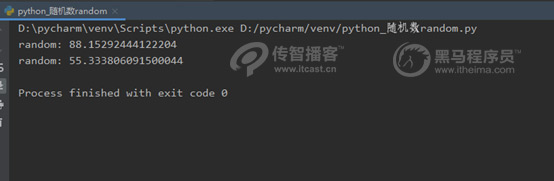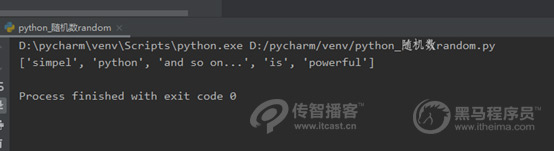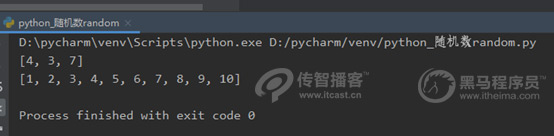# Python随机函数random用法介绍[python培训]

Python中的 random模块用于生成随机数，它提供了很多函数。接下来，针对常见的随机数函数进行讲解，具体如下

1. random.random()

import random

# 生成一个随机数

print("random():", random.random())

# 生成第二个随机数

print("random():", random.random())2. random.uniform(a,b)

import random

print("random:", random.uniform(50, 100))

print("random:", random.uniform(100, 50))3. random.randint(a,b)

import random

# 生成一个随机数N，N的取值范围为12<=N<=20

print(random.randint(12, 20))

# 生成的随机数为N，N 的结果永远为20

print(random.randint(20, 20))

# print(random.randint(20, 10))

# 该语句是错误语句，下限a必须小于上线b4、random.randrange([start], stop[, step])

import random

print(random.randrange(10, 100, 2))5. random.choice(sequence)

random.choice("学习 python")

random.choice(["JGood", , "is", "a", , "handsome", "boy"])

random.choice(("Tuple", , "List", "Dict"))

6、random.shuffle(X[,random])

import random

demo_list = ["python", "is", "powerful", "simpel", "and so on..."]

random.shuffle(demo_list)

print(demo_list)7、random.sample(squence, K)

import random

list_num =[1, 2, 3, 4, 5, 6, 7, 8, 9, 10]

# 定义slice为碎随机在list_num中获取的3个元素

slice = random.sample(list_num, 3)

print(slice)

# 原有的序列没有发生改变

print(list_num)## 相关阅读基础班入门课程限时免费

申请试学名额

基础班入门课程限时免费

申请试学名额

基础班入门课程限时免费

申请试学名额

基础班入门课程限时免费

申请试学名额

基础班入门课程限时免费

申请试学名额

基础班入门课程限时免费

申请试学名额

基础班入门课程限时免费

申请试学名额

基础班入门课程限时免费

申请试学名额

基础班入门课程限时免费

申请试学名额

基础班入门课程限时免费

申请试学名额

基础班入门课程限时免费

申请试学名额

基础班入门课程限时免费

申请试学名额

基础班入门课程限时免费

申请试学名额